03 Apr

After I released Pico Racer, lots of people have thought it looks nice and is an achievement. I don’t think it does anything special or pushes the limits of the Pico-8. Here I try to explain the small tricks I used in the game. If you want a general tutorial about pseudo 3D racers in general then please check out Louis Gorenfeld’s excellent page about the subject, I try to focus on Pico-8 specific stuff.

Usually, with pseudo 3D racers, the road is drawn using a simple trick: there is a full screen graphic of a straight road with perspective and every horizontal line gets shifted left or right, more the further down the road the current horizontal line is. This makes the road look like it’s curving. Palette tricks are used to create the familiar stripes that give an illusion of motion.

With Pico-8, there is no space for a precalculated road asset but we can draw scaled sprites instead. The road in Pico Racer is simply a series of one pixel high sprites scaled by distance, one for both track edges and two used to draw the road surface. The road is a true textured plane instead of a distorted image. Curves works similarly to the above classic method.

Additionally, when rendering the road (from bottom of the screen to the middle of the screen) we iterate along the track data. This is very approximate because we just take the screen Y, calculate the Z and figure out which part of the track the horizontal line belongs to. A more accurate way would be to iterate Z instead of the screen space Y and when Z projected to the screen gives a different screen Y coordinate, we would draw the horizontal line. But going along Y gives a good enough effect, the player will get a good enough hint what is coming towards him.

The road X position on screen is stored for each Y coordinate which we will use later to render the sprites correctly along the road. Note: this gives a slightly crude motion to sprites when they are far away because the X offset are stored per each screen Y coordinate instead of Z. As long as the action is fast, the player will not notice anything.

The road texture graphic used per line is selected so that the further away the line is, the lighter the colors in the texture. Dithering is used to mask the exact position where the texture changes.One textureMultiple texturesDithered textures

This is done because of two reasons: in nature, colors get lighter the further away something is (actually, tint to sky blue beacuse there is air between you and the faraway object) and also so that the road sides and lane markings won’t strobe as much.Lots of flickerLess flicker

## Sprite rendering

Sprites are rendered basically using the same idea as for the horizontal road lines. First, Z is used to determine the zoom factor. Secondly, sprite X position (on screen) is divided by Z and the road offset for the sprite screen Y coordinate is used to offset the sprites so that they are arranged along the road.

### Car rendering and sprite perspective

There is one very important and unavoidable problem with sprites and 3D: the sprites always face the camera. This is not a problem with orthogonal 2D projection, since there is only one way the camera looks at. Everything is on one flat plane. But with a perspective projection the sprites start to look like they are always rotated to face the camera unless you take the time and draw multiple versions of the sprite from different angles. This is how Origin’s Wing Commander and Lucasarts’ Their Finest Hour work. But this takes a lot of effort and more importantly: it needs a lot of space for the sprites.

In Pico Racer, sprite perspective is handled so that most sprites (trees, warning signs) have no perspective. This works reasonably well due to two facts: the objects are two-dimensional (warning signs) AND they are always located to the sides so that the angle to them would be pretty much the same at all times. Only the player car and the opponent cars have visible perspective, since that’s what you look at the most. Also, the cars (especially the player car) have a lot of sideways motion which would immediately make the cars’ perspective look odd.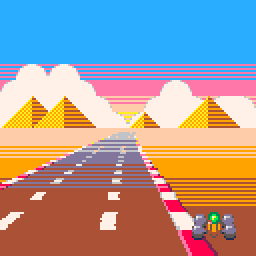No perspective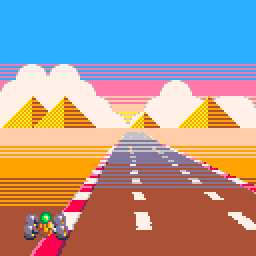Sprite perspective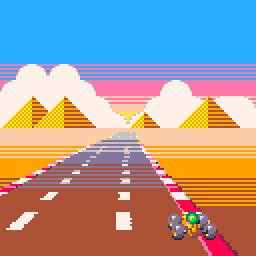Full perspective

The cars use two tricks to fake a convincing perspective: firstly, they have multiple versions of the sprites. Secondly, the cars are built of a number of separate sprites located at slightly different distances. I borrowed this idea from Lankhor’s Vroom and it works well with cars that have no flat sides and instead have clear “sections” – just like F1 cars have. The cars have the rear sprite, the front sprite and a middle section. When the car moves sideways, in addition to showing the sprite from an angle, the rear wheels have some sideways motion against with respect to the front wheels. And, while not a perspective thing, the front section is moved sideways when the player turns left or right, when the road has a curve or when the car bounces giving the illusion the car is yawing and pitching.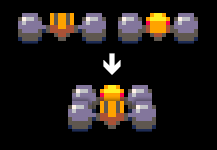Parts of the car

## Night levels and color fades

The color fades in Pico Racer are done using the palette mapping feature in Pico-8 and a few lookup tables. Basically, the tables tell which color to use for each of the 16 colors at a set brightness. Hand picking the colors gives the possibility (actually, with the fixed palette the fade will be tinted) to tint the fades so that they mimic sunset and so on. The road, sky and the sprites use a different lookup table each so that e.g. the road has markings visible even at pitch black and the sky and the horizon have a slightly different fade curve (because nature works like that).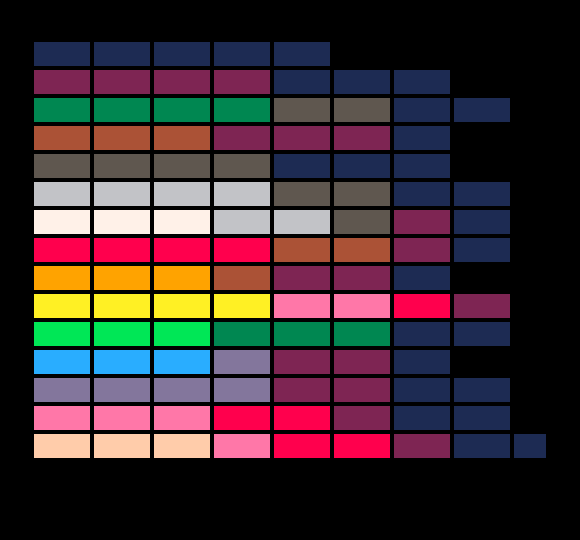Palette lookup table

It all works something like this:

```PALETTE={
{1,1,1,1,2,2,2,0},
...
}

FOR C=1,15 DO
-- ALL COLORS WITH VALUE C ARE NOW DRAWN
PAL(C, PALETTE[C][LEVEL])
END
END```

On night levels, the cars are have rear lights drawn after it is dark enough. They are simply two extra sprites drawn without using the fade lookup palette.

## Pico-8 performance

The Pico-8 is more than enough to do all the math and rendering in one frame (30 FPS). In fact, only the sprites rendering seems to be a bottleneck. Mainly, when sprites are zoomed, their area grows exponentially and the area is used to calculate how long Pico-8 takes to draw the sprite. Sometimes even large but fully clipped (i.e. outside the screen or the clip rectangle) sprites slow everything down. I found the combined area of the sprites is more important than the number of sprites.

In Pico Racer, I capped sprites so that they never zoom larger than 100 %. This has minimal effect on visuals, although you will easily notice it if you know it’s happening. Since e.g. the cars suddenly stop growing as they get closer to the camera, your brain thinks the cars in fact start shrinking. Likewise, since there is a short range where all the cars are drawn equally large, a car a bit further away looks larger than a car closer to the camera, everything because your brain expects things further down the road to be a bit smaller.

As for performance, limiting sprite size gives a big performance boost since as the sprites come very close to the camera and they get larger and larger, they very quickly get zoomed 200 %, 300 %, 600 % and so on. Further away they are just a dozen of pixels for a very long distance.

Since the area of sprites is the main contributing factor, this makes rendering the road quite efficient because every horizontal line is just one pixel high even though there are four sprites per horizontal line and 64 lines total.

25 Jul

Note: There’s now a patch for SDL that makes the same possible on older Androids as well.

The Android NDK makes it possible to use SDL to code Android apps. The aliens.c example bundled with the Android SDL port is fine and dandy except it reads data from /sdcard/data and it has to be pushed manually on the device. A nicer approach is to use the standard SDL way to load data from weird sources: with the SDL_RWops struct. We can use the Android AssetManager object to read data from the APK file (the assets directory in the project), Android 2.3 comes with <android/asset_manager.h> that has the needed helper NDK stuff.

Now, the SDL example is for Android 1.5 (or 1.6, I forget) which means it doesn’t use NativeActivity but instead rolls its own Java wrapper. That means we don’t have simple access to the asset manager — NativeActivity makes this easy because it calls the native code with all the useful stuff ready in a struct android_app — so we have to look it up ourselves.

In short:

1. Using NativeActivity, it’s in struct android_app passed to android_main()

2. If struct android_app is not available (as in the SDL example), store JNI environment in a global variable in the SDL startup code and use it in your code to get AssetManager

2. Use AAsset_RWFromAsset() defined below to get an SDL_RWops to a file inside the assets directory

We have to modify the SDL startup method defined in the library source code:

### SDL_android_main.cpp (line 19-ish)

``````JNIEnv *g_env;

extern "C" void Java_org_libsdl_app_SDLActivity_nativeInit(JNIEnv* env, jclass cls, jobject obj)
{
// Store the environment for later use.
g_env = env;

/* This interface could expand with ABI negotiation, calbacks, etc. */
SDL_Android_Init(env, cls);

/* Run the application code! */
int status;
char *argv;
argv = strdup("SDL_app");
argv = NULL;
status = SDL_main(1, argv);

/* We exit here for consistency with other platforms. */
exit(status);
}``````

### main.c

Note: fatal() is a macro that invokes the Android logging interfaces, much like the LOGE() macro.

``````#include "android_rwops.h"
#include <android/asset_manager_jni.h>
#include <jni.h>

// g_env is set in SDL_android_main.cpp
extern JNIEnv *g_env;

// This function retrieves a static member of SDLActivity called mAssetMgr
// which is initialized in the onCreate() method like so:
// ...
//   mAssetMgr = getAssets();
// ...
// You can also call the getAssets() method from the native code.

AAssetManager * get_asset_manager()
{
jclass sdlClass = (*g_env)->FindClass(g_env, "org/libsdl/app/SDLActivity");

if (sdlClass == 0)
{
return NULL;
}

jfieldID assman = (*g_env)->GetStaticFieldID(g_env, sdlClass,
"mAssetMgr", "Landroid/content/res/AssetManager;");

if (assman == 0)
{
fatal("Could not find mAssetMgr.");
return NULL;
}

jobject assets = (*g_env)->GetStaticObjectField(g_env, sdlClass, assman);

if (assets == 0)
{
fatal("Could not get mAssetMgr.");
return NULL;
}

return AAssetManager_fromJava(g_env, assets);
}

int main(int argc, char **argv)
{
AAssetManager *assets = get_asset_manager();

// You should check the return value, here we assume logo.png is found
SDL_RWops *rw = AAsset_RWFromAsset(assets, "image.bmp");

// Do whatever you want with the rwops

...
}
``````

### android_rwops.h

``````#pragma once

#ifdef _cplusplus
extern "C" {
#endif

#include "SDL_rwops.h"
#include <android/asset_manager.h>

SDL_RWops * AAsset_RWFromAsset(AAssetManager *mgr, const char *filename);

#ifdef _cplusplus
}
#endif``````

### android_rwops.c

``````#include "android_rwops.h"

static SDLCALL long aa_rw_seek(struct SDL_RWops * ops, long offset, int whence)
{
return AAsset_seek((AAsset*)ops->hidden.unknown.data1, offset, whence);
}

static SDLCALL size_t aa_rw_read(struct SDL_RWops * ops, void *ptr, size_t size, size_t maxnum)
{
return AAsset_read((AAsset*)ops->hidden.unknown.data1, ptr, maxnum * size) / size;
}

static SDLCALL int aa_rw_close(struct SDL_RWops * ops)
{
AAsset_close((AAsset*)ops->hidden.unknown.data1);
SDL_FreeRW(ops);

return 0;
}

SDL_RWops * AAsset_RWFromAsset(AAssetManager *mgr, const char *filename)
{
AAsset *asset = AAssetManager_open(mgr, filename, AASSET_MODE_RANDOM);

if (!asset)
return NULL;

SDL_RWops *ops = SDL_AllocRW();

if (!ops)
{
AAsset_close(asset);
return NULL;
}

ops->hidden.unknown.data1 = asset;
ops->write = NULL;
ops->seek = aa_rw_seek;
ops->close = aa_rw_close;

return ops;
}``````
02 Jan

## Introduction

Here’s an audio compression scheme I came up for my tracker project. Generally, I wanted an algorithm that meets these three conditions:

• Has to compress mono audio losslessly
• Has to be simple, should be just one source code file or 500 lines of C code at most
• Should compress 8-bit audio that’s upscaled to 16-bits to near 8 bits per sample

The lossless requirement is obvious and the simplicity gives the benefit of not having to rely on external libraries (e.g. when using something like RAR compression) and not being overkill. The audio data is not huge in any case so the compression ratio doesn’t have to be exceptional. The last requirement is the most important: in klystrack, it is possible to load raw 8-bit audio files, usually originally from the Amiga computer. The native audio format in klystrack is 16-bit, however, so when saving the audio data there always are 8 redundant bits and the file will generally be twice as large as the original file. Also, since the software deals with low-fidelity aesthetics (chiptunes), the audio data might be even lower quality than 8-bit.

While there’s the option of detecting the bit fidelity and only saving the most significant bits, I thought it should be quite simple to devise an algorithm that automatically detects and leaves the redundant bits out while still doing some compression for even 16-bit audio data.

## The algorithm

In short, the algorithm does this:

1. Delta code input data

2. Gray code the data from above

3. Reorder the data from above in bit planes. I.e. first output the least significant bit of all samples, then the next bit for all samples and so forth

4. Run-length encode the above data exploiting the fact you only need to store the distance between bit flips

### Delta encoding

By delta encoding the audio data, we can exploit the fact audio data very often changes just a little between samples and so the generated delta values (the amount of change) need fewer bits to be expressed. However, you can’t store 16-bit data with e.g. 8-bit delta values since it is not assured the change is always small enough to be stored in 8 bits, requiring the use of 16-bit delta values in the worst case.

### Gray encoding

Using gray codes we can minimize the amount of bit flipping that happens (e.g. when a value changes between 7 and 8, where the bits change from 0111 to 1000 — three bits flip even if the value changes by one) to one bit flip between any two neighboring values. In practice, this doesn’t always result in better compression and sometimes it even can hurt the compression ratio.

### Reordering data in bit planes

This step is the key to good compression in our case. Between two neighboring delta values, it’s common that most of the bits are the same and it’s common for one bit to stay completely static for the whole data. So, we take the bits from all samples and sort them so that all significant bits come before the lesser bits. The result is long runs of bits, usually in the most significant bits.

### Run-length encoding

Finally, we take the encoded and reordered data and store the bits using RLE. While RLE usually stores the repeated data and the number of repetitions, we can exploit the fact the data is always zero or one, always changing between runs — only the starting state of the bit needs to be stored. Elias gamma coding is used to store the run-lengths since in most cases the run length is in the range of a few bits (for completely empty bit planes we can use special flags that tell they’re all zero or one) and tend to fall in 1-4 bits. With gamma coding it’s possible to store the number 1 by using one bit, the number two takes three bits.

Also, for some bit planes a raw dump of bits with no compression should be used if compression would result in much longer data than the original. There are also two special codes for the bit planes, in cases where all bits are zero or one (common if the audio data is quiet, i.e. uses only a subrange of the 16-bit resolution) or if the data is offset by a constant.

Depending on implementation the total overhead is around two bits per bit plane (i.e. 32 bits or two bytes for 16-bit audio data), not including the header data describing the compressed data (data length, uncompressed length). Since there’s the possibility of a raw dump of bits, in the worst case (none of the bit planes compress at all), the data size grows only by two bytes. A completely quiet (all zero bits) data will take only two bytes for any length.

## Improvements

My implementation only compresses the whole audio data as one big chunk but this can be easily improved to compress in chunks. This should improve the compression when there are parts where the audio is quiet and most of the bits will be zero.

Stereo audio can be compressed by compressing two chunks of data, one for both channels. The other channel should be delta coded compared to the other channel since in stereo audio the two channels often heavily correlate.

Since the Gray coding may even hurt compression, there should be the option to disable the coding for chunks. This can be simply by brute force, comparing which combination of options produces the shortest compressed data.

## Compression results

The following contains example compression results. The Option column tells different configurations where the input data is delta coded and/or Gray coded. This shows how different preprocessing steps work better for different kinds of input data.

Legend: 0 = no coding before compression, 1 = delta coding, 2 = Gray coding, 3 = delta and Gray coding.

Options Ratio
Sine wave (loud)
0 73.1 %
1 29.6 %
2 67.9 %
3 22.6 %
White noise (loud)
0 100.0 %
1 100.0 %
2 99.8 %
3 100.0 %
Complex wave
0 75.3 %
1 38.2 %
2 69.6 %
3 30.0 %
Options Ratio
Sine wave and noise
0 84.7 %
1 100.0 %
2 80.9 %
3 80.9 %
White noise (quiet)
0 50.0 %
1 100.0 %
2 50.0 %
3 55.9 %
Complex wave (saturated)
0 38.9 %
1 21.9 %
2 37.2 %
3 17.7 %

## Source code

```#include <stdio.h>
#include <stdlib.h>
#include <string.h>
#include <math.h>

typedef unsigned char Uint8;
typedef short int Sint16;
typedef unsigned short int Uint16;
typedef int Sint32;
typedef unsigned int Uint32;

/* bitpack() bit plane codes */
enum
{
BITPACK_STATIC0,
BITPACK_STATIC1,
BITPACK_LITERAL,
BITPACK_RLE
};

/* bitstream writer helpers*/
typedef struct
{
Uint8 *buffer;
Uint32 byte, size_byte; 	// Read/write position counters (byte component)
Uint8 bit, size_bit;		// Read/write position counters (bit component)
} BitPtr;

#define BIT_BLOCK_SIZE 1024 // Bitstream buffer allocation increment size

void bit_init(BitPtr *p, Uint8 *buffer, Uint32 size_bytes, Uint8 size_bits)
{
memset(p, 0, sizeof(*p));
p->buffer = buffer;
p->size_byte = size_bytes;
p->size_bit = size_bits;
}

void bit_deinit(BitPtr *p)
{
free(p->buffer);
memset(p, 0, sizeof(*p));
}

void bit_rewind(BitPtr *p)
{
p->bit = p->byte = 0;
}

void bit_seek(BitPtr *p, Uint32 byte, Uint8 bit)
{
p->byte = byte;
p->bit = bit;
}

void bit_w(BitPtr *p, int bit)
{
if (p->bit == 0 && !(p->byte & (BIT_BLOCK_SIZE - 1)))
{
// If write position modulo BIT_BLOCK_SIZE is zero, allocate a larger buffer
p->buffer = realloc(p->buffer, p->byte + BIT_BLOCK_SIZE);
}

if (bit)
p->buffer[p->byte] |= 1 << p->bit;
else
p->buffer[p->byte] &= ~(1 << p->bit);

++p->bit;

if (p->bit == 8)
{
p->bit = 0;
++p->byte;
}

p->size_byte = p->byte;
p->size_bit = p->bit;
}

int bit_r(BitPtr *p)
{
if (p->size_byte <= p->byte && p->size_bit <= p->bit)
return -1;

int bit = (p->buffer[p->byte] & (1 << p->bit)) != 0;

++p->bit;

if (p->bit == 8)
{
p->bit = 0;
++p->byte;
}

return bit;
}

/* Write Elias gamma coded value (has to be nonzero) */
void bit_wgamma(BitPtr *p, Uint32 value)
{
int l = log2(value);

for (int a=0; a < l; a++)
bit_w(p, 0); //put 0s to indicate how many bits will follow

bit_w(p, 1);      //mark the end of the 0s

for (int a=l-1; a >= 0; a--) //Write the bits as plain binary
{
bit_w(p, value & 1 << a);
}

}

/* Read Elias gamma coded value, zero return value signals read error */
Uint32 bit_rgamma(BitPtr *p)
{
int numberBits = 0;
int bit;
while (!(bit = bit_r(p)))
numberBits++; //keep on reading until we fetch a one...

if (bit < 0) return 0;

Uint32 current = 0;
for (int a=numberBits-1; a >= 0; a--) //Read numberBits bits
{
if ((bit = bit_r(p)))
current |= 1 << a;

if (bit < 0) return 0;
}
current |= 1 << numberBits; //last bit isn't encoded!

return current;
}

Sint32 bit_rbits(BitPtr *p, Uint8 bits)
{
Sint32 rval = 0;

for (int i = 0 ; i < bits ; ++i)
{
int bit = bit_r(p);

if (bit < 0) return -1;

rval |= bit << i;
}

return rval;
}

/* Write bits bits of v */
void bit_wbits(BitPtr *p, Uint32 v, Uint8 bits)
{
for (int i = 0 ; i < bits ; ++i)
{
bit_w(p, v & (1 << i));
}
}

/* Gray and delta encoding helpers */
static inline Uint16 gray(Uint16 v)
{
return v ^ (v >> 1);
}

static inline Uint16 degray(Uint16 v)
{
v ^= (v>>8);
v ^= (v>>4);
v ^= (v>>2);
v ^= (v>>1);
return v;
}

static void delta_encode(Sint16 *buffer, const int n)
{
Sint32 delta = 0;
Sint32 original;
for (int i = 0; i < n; ++i)
{
original = buffer[i];
Sint32 x = original - delta;
buffer[i] = gray(x + 32768); // Gray code can not be negative
delta = original;
}
}

static void delta_decode(Sint16 *buffer, const int n)
{
Sint32 delta = 0;
for (int i = 0; i < n; ++i)
{
Sint32 v = degray(buffer[i]);
buffer[i] = (v - 32768) + delta;
delta = buffer[i];
}
}

/* Compress 16-bit signed data into bitstream, return compressed data size in packed_size (in bits) */
Uint8 * bitpack(const Sint16 *_buffer, const int n, Uint32 *packed_size)
{
BitPtr bp;
bit_init(&bp, NULL, 0, 0);

Sint16 *buffer = malloc(sizeof(Sint16) * n);
memcpy(buffer, _buffer, sizeof(Sint16) * n);

delta_encode(buffer, n);

for (int plane = 0 ; plane < sizeof(*buffer) * 8 ; ++plane)
{
const Sint16 mask = 1 << plane;
int bit = mask & *buffer;

int type = BITPACK_STATIC0 | (bit != 0);

for (int i = 0 ; i < n ; ++i)
if ((buffer[i] & mask) != bit)
{
// Was not all zeros or all ones, needs to compress
type = BITPACK_RLE;
break;
}

Uint32 p_byte = bp.byte;
Uint32 p_bit = bp.bit;

again:

bit_wbits(&bp, type, 2);

switch (type)
{
case BITPACK_LITERAL:
for (int i = 0 ; i < n ; ++i)
break;

case BITPACK_STATIC0:
case BITPACK_STATIC1:
// No data needed, bit plane is all zeros or all ones
break;

case BITPACK_RLE:
{
// Write starting bit state
bit_w(&bp, bit);

Uint32 ctr = 0;

for (int i = 0 ; i < n ; ++i)
{
if (((buffer[i] & mask) == bit))
++ctr;

if ((buffer[i] & mask) != bit)
{
bit_wgamma(&bp, ctr);

ctr = 1;

// Flip the bit (no neighboring bits are the same state)
}
}

if (ctr != 0) bit_wgamma(&bp, ctr);

if ((bp.byte * 8 + bp.bit) - (p_byte * 8 + p_bit) > n + 2)
{
// RLE gave longer data than the original, dump data instead
bit_seek(&bp, p_byte, p_bit);
type = BITPACK_LITERAL;
goto again;
}

}
break;
}

}

free(buffer);

*packed_size = bp.byte * 8 + bp.bit;

return bp.buffer;
}

/* Decompress compressed bitstream into 16-bit signed data, decompress at most packed_size bits
unpacked_size tells the function the data length (important)
*/
Sint16 * bitunpack(const Uint8 *packed_data, const Uint32 packed_size, Uint32 unpacked_size)
{
BitPtr bp;
bit_init(&bp, (Uint8*)packed_data, packed_size / 8, packed_size & 7);

Sint16 *buffer = calloc(unpacked_size, sizeof(Sint16));

for (int plane = 0 ; plane < sizeof(*buffer) * 8 ; ++plane)
{
const Sint16 mask = 1 << plane;

Sint32 type = bit_rbits(&bp, 2);

if (type < 0) goto read_error;

switch (type)
{
case BITPACK_LITERAL:
for (int i = 0 ; i < unpacked_size ; ++i)
{
int bit = bit_r(&bp);
if (bit < 0) goto read_error;
}
break;

case BITPACK_STATIC0:
// Data bits are zero by default, no action needed
break;

case BITPACK_STATIC1:
// Fill bit plane with set/unset bit
for (int i = 0 ; i < unpacked_size ; ++i)
break;

case BITPACK_RLE:
{
// Read the starting bit status
int bit = bit_r(&bp);

if (bit < 0) goto read_error;

if (bit) bit = mask; else bit = 0;

buffer |= bit;

for (int i = 0 ; i < unpacked_size ; )
{
Uint32 ctr = bit_rgamma(&bp);

if (ctr == 0) goto read_error;

for (; i < unpacked_size && ctr ; ++i, --ctr)
buffer[i] |= bit;

// Flip the bit (neighboring bits are always different)
}
}
break;
}
}

delta_decode(buffer, unpacked_size);

if (0)
{
free(buffer);
return NULL;
}

return buffer;
}

/* Generate test data, 0 is a sine wave and 1 generates noise */
void getdata(Sint16 *buffer, const int n, int t)
{
switch (t)
{
case 0:
for (int i = 0 ; i < n ; ++i)
{
buffer[i] = (((int)(sin((float)i / 1000 * 3.141 * 2) * 20000)));
}

break;

case 1:
for (int i = 0 ; i < n ; ++i)
buffer[i] = (rand() * 4);

break;
}
}

int main(int argc, char **argv)
{
const int n = 100000;

Sint16 *b = malloc(sizeof(Sint16) * n);
Uint32 packed_size;

getdata(b, n, 0);

Uint8 * data = bitpack(b, n, &packed_size);
Sint16 * udata = bitunpack(data, packed_size, n);

if (udata == NULL || memcmp(b, udata, sizeof(Sint16) * n) != 0)
{
printf("Upack failed\n");
}
else
{
printf("Compression ratio %.1f %%\n", (float)100 * packed_size / (n * sizeof(Sint16) * 8));

}

free(data);
free(udata);
free(b);

return 0;
}
```
28 Feb

My new weapon of choice. libconfig is a configuration file parser that supports arrays, named and typed members, selection by path (e.g. cfg.users..name) and more. That is, it has basically all the useful (as in 80% of cases) features of XML and none of the bad. There’s a minimal but well defined structure that will work for most situations and that can be used to enforce e.g. all array items having to be of the same type. There’s no overkill markup so it’s easy to read and write by humans. The library can also write the settings tree into a text file.

The configuration files look like this:

```screen = { width = 300; height = 200; }
users = ( { name = "Torgo"; items = [ "Item 1", "Item 2" ]; } );```

And in C you would do something like this:

```int screen_height = 100;
const char *name;
config_init(&cfg);
if (!config_lookup_int(&cfg, "screen.height", &screen_height))
puts("Using default screen height");
if (config_lookup_string(&cfg, "users..name", &name))
puts(name);
config_destroy(&cfg);```

You can also iterate the setting tree without the path for easier array or tree traversal. In all, I would say it involves less work compared to any XML library, especially in C. I like to think it’s a good example of software designed by the same guy who also uses it and not by some external committee.

22 Sep

… Or, How to Procrastinate Productively.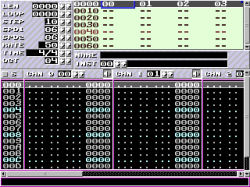I decided to make one of my current projects open source and post them on Google Code just for fun. The project is a tool chain that I’m using to remake Thrust. In reality, I decided to divide the project into two separate projects: the actual game engine (called klystron) and related tools, and a music editor that uses the engine.

Here are two videos I made a while ago that demonstrate the engine. The first is the music editor (called klystrack) — it’s much less ugly at the moment but the sound synthesis is the same, and that’s what matters:

The sound engine (“Cyd”) is basically a very simple software synthesizer with capabilities comparable to the SID or any 8-bit machine from the 80s. The editor is a fairly standard tracker, much like GoatTracker.

The graphics half of the engine is basically a wrapper around a quite fast collision detection system (pixel-accurate, or it wouldn’t be much good for a thrustlike) built on SDL. It also does background collisions and drawing as well. As you may have guessed, the whole point is to provide a limited but still helpful set of routines that are useful for creating 2D games not unlike what video games were in 1991.

And, here’s a proof I’m actually working on the actual game (the sound effects are created in real time by the sound engine):

A note on Google Code: it’s rather nice. It provides the standard open source development stuff like source control an such but I really like how clean and hassle-free it is. Adding a project takes a minute and after that it’s simply coding and some quick documentation on the project wiki. The project wiki is good example of how simple but elegant the system is: the wiki pages actually exists inside the source control as files, just like your source code.

Go check Google Code out and while you’re at it, contribute on my projects. :)